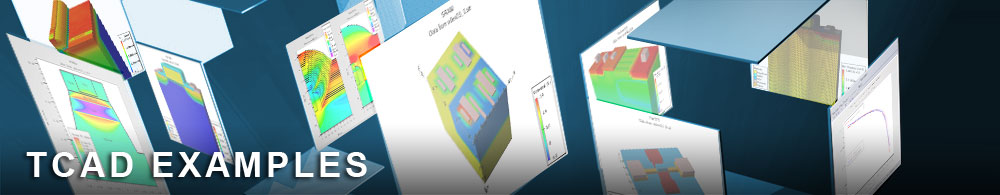Emission Spectrum Analysis

## laserex02.in : Emission Spectrum Analysis

Requires: Blaze/Laser
Minimum Versions: Atlas 5.28.1.R

This example demonstrates simulation of a buried heterostructure InP/InGaAsP laser diode. A physically based frequency dependent optical gain model is used in this example to calculate light emission spectrum. The example shows:

• Laser structure definition using Atlas syntax
• Setting up the device models and initial biasing
• Activating LASER module of Atlas
• Mesh definition for solving the Helmholtz wave equation
• Definition of laser physical models and their parameters including the frequency dependent optical gain model
• Calculation of laser electrical and optical characteristics

This example illustrates how Atlas can be used to simulate spectral characteristics. The spectral characteristics refer to the longitudinal laser spectrum since LASER takes into account only the fundamental transverse mode solution. It is known from the theory that the longitudinal laser spectrum depends on the optical gain, spontaneous emission spectrum, and the cavity length in the third dimension (or Z-direction). A shorter cavity length leads to a wider energy spacing between adjacent longitudinal modes, and so fewer longitudinal modes will be actually lasing. From the computational point of view the situation of the nearly single mode laser operation is very desirable because each lasing mode considered imposes an additional computational burden.

For illustration purposes the structure under consideration is the same as in the previous example in this section. We refer to that example regarding the laser structure definition, mesh, material and physical models specification.

The difference between this and the previous simulation is that physically based optical gain modes are activated in LASER simulation using the syntax models lmodes gainmod=1 . Gainmod=1 sets the frequency dependent model. The parameters las_einit las_efinal are used to specify the photon energy range for spectral analysis, and the cavity_length parameter is set to 50 microns. The latter value is rather small but it allows us to demonstrate the spectral analysis within a comparatively short run time.

As in the previous example the additional variables specified in the output statement will be saved in the output structure file. These include the conduction and valence band potentials for analyzing the energy band diagram, total, SRH, Auger, and radiative recombination rates, and current flowlines.

During simulation the IV data, light power, and total optical gain data is stored in the log file. The laser spectra (gain spectra and photon density spectra) are stored in separate log files for each bias condition. The name of the spectrum log file is specified using the parameter spec.name in the models statment in the LASER portion of the input file. The following results are displayed in TonyPlot:

• Total light output power versus current
• Gain spectra for different laser biases (currents) below and above lasing threshold
• Light output spectrum below and above lasing threshold

For comparison, the light output power vs diode current is shown along with the similar data obtained in the previous example. The gain saturation effect is clearly seen after laser threshold. Only a few of the longitudinal modes are actually lasing and the light output spectrum is practically the same after the lasing threshold.

To load and run this example, select the Load button in DeckBuild > Examples. This will copy the input file and any support files to your current working directory. Select the Run button in DeckBuild to execute the example.